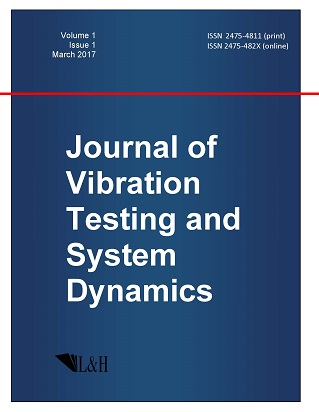ISSN: 2475-4811 (print)
ISSN: 2475-482X (online)
Journal of Vibration Testing and System Dynamics

C. Steve Suh (editor), Pawel Olejnik (editor),

Xianguo Tuo (editor)

Pawel Olejnik (editor)

Lodz University of Technology, Poland

Email: pawel.olejnik@p.lodz.pl

C. Steve Suh (editor)

Texas A&M University, USA

Email: ssuh@tamu.edu

Xiangguo Tuo (editor)

Sichuan University of Science and Engineering, China

Email: tuoxianguo@suse.edu.cn

Existence and Exponential Stability for Neutral Impulsive Stochastic Integrodifferential Equations with Fractional Brownian Motion Driven by Poisson Jumps

Journal of Vibration Testing and System Dynamics 4(4) (2020) 311--324 | DOI:10.5890/JVTSD.2020.12.002

K. Ramkumar , K. Ravikumar, A. Anguraj

Department of Mathematics, PSG College of Arts and Science, Coimbatore, 641 014, India

Abstract

In this paper, we establish the results on existence and uniqueness of mild solution of neutral impulsive stochastic integrodifferential equations with fractional Brownian motion driven by Poisson jumps in a Hilbert space. Further, by using an impulsive integral inequality, we study the new sufficient conditions that ensure the exponential stability of mild solution in the mean square moment by utilizing the fractional power of operators and the semigroup theory.

References

1.   Anguraj, A. and Vinodkumar, A. (2009), Existence, uniqueness and stability results of impulsive stochastic semi linear neutral functional differential equations with infinite delays, Electron. J. Qual. Theory Differ. Equ., 67, 1-13.
2.   Boufoussi, B. and Hajji, S. (2010), Successive approximation of neutral functional stochastic differential equations with jumps, Stat. Probab. Lett., 80, 324-332.
3.   Cui, J. and Yan, L. (2012), Successive approximation of neutral stochastic evolution equations with infinite delay and Poisson jumps, Appl. Math. Comput., 218, 6776-6784.
4.   Da Prato, G. and Zabczyk, J. (2014), Stochastic Equations in Infinite Dimensions, Cambridge University Press: Cambridge.
5.   Diop, M.A., Ezzinbi, K., and Lo, M. (2014), Existence and exponential stability for some stochastic neutral partial functional integrodifferential equations, Random. Oper. Stoch. Equ., 22(2), 73-83.
6.   Caraballo, T., Real, J., and Taniguchi, T. (2007), The exponential stability of neutral stochastic delay partial differential equations, Discrete Cont. Dyn. Syst., 18, 295-313.
7.   Pazy, A. (1983), Semigroups of Linear Operators and Applications to Partial Differential Equations, Applied Mathematical Sciences, Vol. 44, Springer: New York.
8.   Anguraj, A., Ramkumar, K., and Elsayed, E.M. (2020), Existence, uniqueness and stability of impulsive stochastic partial neutral functional differential equations with infinite delays driven by a fractional Brownian motion, Discontinuity, Nonlinearity, and Complexity, 9(2), 327-337.
9.   Diop, M.A., Ezzinbi, K., and Lo, M. (2014), Asymptotic stability of impulsive stochastic partial integrodifferential equations with delays, Stochastics Int. J. Probab. Stochastic Process, 86(4), 696-706.
10.   Hu, L. and Ren, Y. (2010), Existence results for impulsive neutral stochastic functional integro-differential equations with infinite delays, Acta Appl. Math., 111, 303-317.
11.   Yang, H. and Jiang, F. (2013), Exponential stability of mild solutions to impulsive stochastic neutral partial differential equations with memory, Adv. Differ. Equ., Article ID 148.
12.   Diop, M.A. and Zene, M.M. (2016), On the asymptotic stability of impulsive neutral stochastic partial integrodifferential equations with variable delays and Poisson jumps, Afr. Mat., 27(1), 215-228.
13.   Anguraj, A., Banupriya, K., Dumitru, B., and Vinodkumar, A. (2018), On neutral impulsive stochastic differential equations with Poisson jumps, Advances in Difference Equations, 290.
14.   Vanterler da, J., Sousa, C., Kucche, K.D., and Capelas de O.E. (2019), Stability of $\psi$-Hilfer impulsive fractional differential equation, Appl. Math. Lett., 88, 73-90.
15.   Sun, M. and Xu, M. (2017), Exponential stability and interval stability of a class of stochastic hybrid systems driven by both Brownian motion and Poisson jumps, Physica A, 487, 58-73.
16.   Luo, J. and Liu, K. (2008), Stability of infinite dimensional stochastic evolution equations with memory and Markovian jumps, Stochastic Process. Appl., 118, 864-895.
17.   Cui, J., Yan, L., and Sun, X. (2011), Exponential stability for neutral stochastic partial differential equations with delays and Poisson jumps, Statist. Probab. Lett., 81, 1970-1977.
18.   Anguraj, A. and Ravikumar, K. (2019), Existence and stability results for impulsive stochastic functional integrodifferential equations with Poisson jumps, Journal of Applied Nonlinear Dynamics, 8(3), 407-417.
19.   Anguraj, A. and Ravikumar, K. (2020), Existence and stability of impulsive stochastic partial neutral functional differential equations with infinite delays and Poisson jumps, Discontinuity, Nonlinearity, and Complexity, 9(2), 245-255.
20.   Dhanalakshmi, K. and Balasubramaniam, P. (2019), Stability result of higher-order fractional neutral stochastic differential system with infinite delay driven by Poisson jumps and Rosenblatt process, Stochastic Analysis and Applications, 38, 352-372.
21.   Ma, Y.K., Arthi, G., and Marshal, A.S. (2018), Exponential behavior of neutral stochastic integrodifferential equations with fractional Brownian motion and impulsive effects, Advances in Difference Equations, 110.